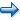# Changing Response Keys

By default, the IAT uses the E and I keys to indicate a left and right response. The response keys are defined using the <trial> element, which is rseponsible for presenting stimuli and gathering responses. The IAT has six different <trial> elements. The first two, shown below, present stimuli from attribute A and attribute B respectively:

```<trial attributea>
/ validresponse = ("E", "I")
/ correctresponse = ("E")
/ stimulusframes = [1 = attributeA, errorReminder]
/ posttrialpause = parameters.ISI
</trial>

<trial attributeb>
/ validresponse = ("E", "I")
/ correctresponse = ("I")
/ stimulusframes = [1 = attributeB, errorReminder]
/ posttrialpause = parameters.ISI
</trial>
```

The validresponse command defines two permissible responses for either trial, "E" and "I". The correctresponse defines which of these responses is considered correct for purposes of scoring and error feedback. For attribute A, the "E" key is always correct. For attribute B, the "I" key is correct. You can change the keys used for responding by replacing "E" and "I" with whatever character you like. If you are using another input device such as a response box, you can replace these with the numeric values corresponding to the buttons.

The remaining four trials present targets A and B. Recall that the IAT presents the targets with both compatible and incompatible attribute pairings, so there are two trials defined for each target category that vary in whether "E" or "I" is considered the correct response. Again, the particular keys used can be modified as they were with the attribute trials.

```<trial targetBleft>
/ validresponse = ("E", "I")
/ correctresponse = ("E")
/ stimulusframes = [1 = targetB, errorReminder]
/ posttrialpause = parameters.ISI
</trial>

<trial targetBright>
/ validresponse = ("E", "I")
/ correctresponse = ("I")
/ stimulusframes = [1 = targetB, errorReminder]
/ posttrialpause = parameters.ISI
</trial>

<trial targetAleft>
/ validresponse = ("E", "I")
/ correctresponse = ("E")
/ stimulusframes = [1 = targetA, errorReminder]
/ posttrialpause = parameters.ISI
</trial>

<trial targetAright>
/ validresponse = ("E", "I")
/ correctresponse = ("I")
/ stimulusframes = [1 = targetA, errorReminder]
/ posttrialpause = parameters.ISI
</trial>
```

The tutorial is now complete! You can run the IAT by selecting the "Run" command on the "Experiment" menu.Modifying Instructions Back to Overview